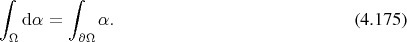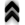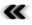### 4.7 Integration

So far diﬀerential structures have been explored which have proved essential in the development of physical sciences. Diﬀerential descriptions are, however, not the only descriptions available. In the following the concepts for the dual operation of diﬀerentiation are outlined, which not only provide diﬀerent descriptions as in Section 5.6, but also inspire diﬀerent methodologies. It requires a few very basic deﬁnitions on which to build.

Deﬁnition 87 (Borel set) A set which is obtained from open sets of a topological space (Deﬁnition 29) using a countable number of unions, intersections and complements is called a Borel set.

Another term is introduced in order to simplify the subsequent Deﬁnition 89.

Deﬁnition 88 (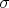-algebra) A non-empty collection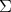of subsets of a set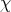such that it is closed under countably many unions, intersections, or complements of elements is called a-algebra.

In the case of dealing with topological spaces a particular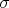-algebra, which contains all open sets, is of special interest.

Deﬁnition 89 (Borel-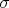-algebra) The set of all Borel sets (Deﬁnition 87) is the Borel-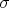-algebra.

Deﬁnition 90 (Measurable space) The pair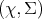of a set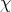along with a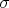-algebra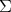is called a measurable space.

Deﬁnition 91 (Signed measure) A mapping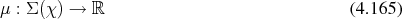assigning a real number to any subset contained in the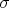-algebra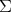(Deﬁnition 88) over the set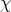, such that for any collection of disjoint sets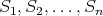in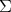it holds that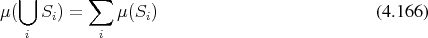is called a signed measure.

When the mapping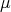is restricted to values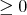, it is called simply a measure.

Deﬁnition 92 (Measure space) A measurable space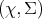(Deﬁnition 90) along with a measure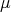forming the triple of a set, a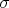-algebra (Deﬁnition 88) and a measure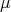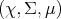form a measure space.

Providing a measure on a Borel-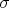-algebra (Deﬁnition 89) is an essential step of deﬁning integration on topological spaces (Deﬁnition 29) such as manifolds (Deﬁnition 35).

The Lebesgue measure is a particular choice for a measure which is related to an interval of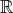in the form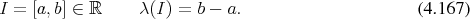The generalization to subsets of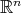is then simply obtained by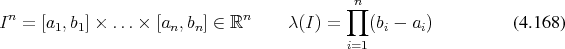Deﬁnition 93 (Almost everywhere) A property is said to apply almost everywhere in a measure space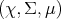, if the complement of the set, where the property does not hold, has measure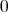.

Using a measure it is possible to introduce the notion of integration of functions. The ﬁrst step is to examine functions of the form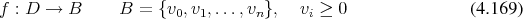resulting in only a limited, ﬁnite number of results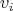, also called step functions. An integral of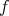using a measure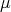is then obtained by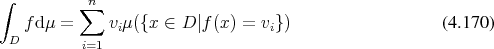Deﬁnition 94 (Integral of a function) Using the step functions, the integral of functions of the form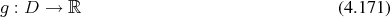is then deﬁned as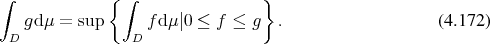It follows from this deﬁnition that the integral does not change its value as long as only function values associated with sets of measure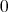are changed.

The integral obtained by using a Lebesgue measure is called the Lebesgue integral, which while not being the most general notion of integration available, shall be suﬃcient for matters at hand.

In the context of manifolds the Borel-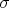-algebra (Deﬁnition 89) can be presented very powerfully by the concept of chains , which provide convenient methods of expression for operations on the integration domain.

It should be noted that the integration domain and the domain of the function being integrated must agree for the integral to be well deﬁned (Deﬁnition 94). An example of such agreement, which may still be embedded in a greater structure of a manifold (Deﬁnition 35) is given next.

Deﬁnition 95 (Curve integrals) The integral of a-form (Deﬁnition 50) along a segment of a curve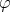(Deﬁnition 46) connecting two points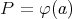andis simply deﬁned as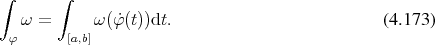One of the most important properties of integration is that operations on the integration domain may be mapped to the integrand by pullback (Deﬁnition 44).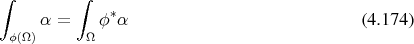This general case specializes to a particularly important case for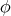being the boundary operation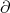called Stokes’ theorem, which asserts that when integrating a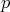-form on a-integration domain it follows that